UPSC  >  NCERT Solutions: Triangles (Exercise 7.2)

# Triangles (Exercise 7.2) NCERT Solutions - NCERT Textbooks (Class 6 to Class 12) - UPSC

Q1. In an isosceles triangle ABC, with AB = AC, the bisectors of ∠ B and ∠ C intersect each other at O. Join A to O. Show that:
(i) OB = OC
(ii) AO bisects ∠ A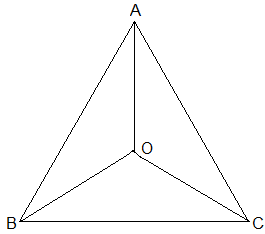Ans:
Given:
AB = AC and
the bisectors of ∠B and ∠C intersect each other at O
(i) Since ABC is an isosceles with AB = AC,
∠B = ∠C
½ ∠B = ½ ∠C
⇒ ∠OBC = ∠OCB (Angle bisectors)
∴ OB = OC (Side opposite to the equal angles are equal.)
(ii) In ΔAOB and ΔAOC,
AB = AC (Given in the question)
AO = AO (Common arm)
OB = OC (As Proved Already)
So, ΔAOB ≅ ΔAOC by SSS congruence condition.
BAO = CAO (by CPCT)
Thus, AO bisects ∠A.

Q2. In ΔABC, AD is the perpendicular bisector of BC (see Fig.). Show that ΔABC is an isosceles triangle in which AB = AC.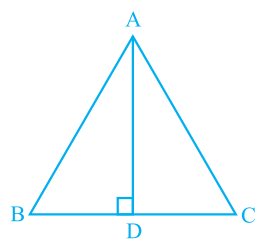Ans: It is given that AD is the perpendicular bisector of BC
To prove:
AB = AC
Proof:
BD = CD (Since AD is the perpendicular bisector)
Thus,
AB = AC (by CPCT)

Q3. ABC is an isosceles triangle in which altitudes BE and CF are drawn to equal sides AC and AB respectively (see Fig.). Show that these altitudes are equal.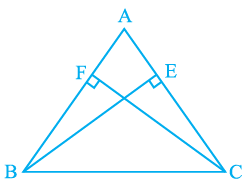Ans:
Given:
(i) BE and CF are altitudes.
(ii) AC = AB
To prove:
BE = CF
Proof:
Triangles ΔAEB and ΔAFC are similar by AAS congruency since
∠A = ∠A (It is the common arm)
∠AEB = ∠AFC (They are right angles)
AB = AC (Given in the question)
∴ ΔAEB ≅ ΔAFC and so, BE = CF (by CPCT).

Q4. ABC is a triangle in which altitudes BE and CF to sides AC and AB are equal (see Fig). Show that
(i) ∆ ABE ≅ ∆ ACF
(ii) AB = AC, i.e., ABC is an isosceles triangle.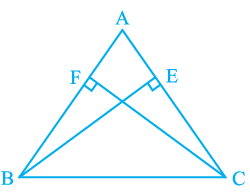Ans: It is given that BE = CF
(i) In ΔABE and ΔACF,
∠A = ∠A (It is the common angle)
∠AEB = ∠AFC (They are right angles)
BE = CF (Given in the question)
∴ ΔABE ≅ ΔACF by AAS congruency condition.
(ii) AB = AC by CPCT and so, ABC is an isosceles triangle.

Q5. ABC and DBC are two isosceles triangles on the same base BC (see Fig). Show that ∠ ABD = ∠ ACD.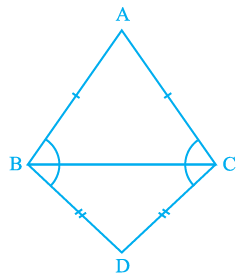Ans: In the question, it is given that ABC and DBC are two isosceles triangles.
We will have to show that ∠ABD = ∠ACD
Proof:
Triangles ΔABD and ΔACD are similar by SSS congruency since
AB = AC (Since ABC is an isosceles triangle)
BD = CD (Since BCD is an isosceles triangle)
So, ΔABD ≅ ΔACD.
∴ ∠ABD = ∠ACD by CPCT.

Q6. ∆ABC is an isosceles triangle in which AB = AC. Side BA is produced to D such that AD = AB (see Fig). Show that ∠ BCD is a right angle.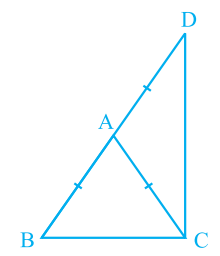Ans: It is given that AB = AC and AD = AB
We will have to now prove ∠BCD is a right angle.
Proof:
Consider ΔABC,
AB = AC (It is given in the question)
Also, ∠ACB = ∠ABC (They are angles opposite to the equal sides and so, they are equal)
Now, consider ΔACD,
Also, ∠ADC = ∠ACD (They are angles opposite to the equal sides and so, they are equal)
Now,
In ΔABC,
∠CAB + ∠ACB + ∠ABC = 180°
So, ∠CAB + 2∠ACB = 180°
⇒ ∠CAB = 180° – 2∠ACB — (i)
∠CAD = 180° – 2∠ACD — (ii)
also,
∠CAB + ∠CAD = 180° (BD is a straight line.)
Adding (i) and (ii) we get,
∠CAB + ∠CAD = 180° – 2∠ACB+180° – 2∠ACD
⇒ 180° = 360° – 2∠ACB-2∠ACD
⇒ 2(∠ACB+∠ACD) = 180°
⇒ ∠BCD = 90°

Q7. ABC is a right angled triangle in which ∠ A = 90° and AB = AC. Find ∠ B and ∠ C.
Ans: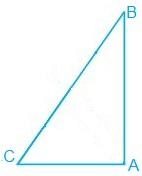In the question, it is given that

∠A = 90° and AB = AC
AB = AC
⇒ ∠B = ∠C (They are angles opposite to the equal sides and so, they are equal)
Now,
∠A+∠B+∠C = 180° (Since the sum of the interior angles of the triangle)
∴ 90° + 2∠B = 180°
⇒ 2∠B = 90°
⇒ ∠B = 45°
So, ∠B = ∠C = 45°

Q8. Show that the angles of an equilateral triangle are 60° each.
Ans: Let ABC be an equilateral triangle as shown below: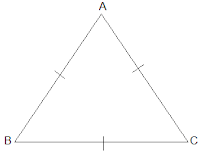Here, BC = AC = AB (Since the length of all sides is same)
⇒ ∠A = ∠B =∠C (Sides opposite to the equal angles are equal.)
Also, we know that
∠A+∠B+∠C = 180°
⇒ 3∠A = 180°
⇒ ∠A = 60°
∴ ∠A = ∠B = ∠C = 60°
So, the angles of an equilateral triangle are always 60° each.

The document Triangles (Exercise 7.2) NCERT Solutions | NCERT Textbooks (Class 6 to Class 12) - UPSC is a part of the UPSC Course NCERT Textbooks (Class 6 to Class 12).
All you need of UPSC at this link: UPSC

## NCERT Textbooks (Class 6 to Class 12)

1402 docs|679 tests

## FAQs on Triangles (Exercise 7.2) NCERT Solutions - NCERT Textbooks (Class 6 to Class 12) - UPSC

 1. What are the different types of triangles?Ans. There are three main types of triangles: equilateral, isosceles, and scalene. An equilateral triangle has all sides and angles equal, an isosceles triangle has two sides and angles equal, and a scalene triangle has no sides or angles equal.
 2. How do I find the area of a triangle?Ans. The area of a triangle can be found using the formula: Area = (base * height) / 2. The base is the length of the triangle's bottom side, and the height is the perpendicular distance from the base to the opposite vertex.
 3. How do I determine if three given sides form a triangle?Ans. To determine if three given sides form a triangle, you can apply the triangle inequality theorem. It states that the sum of the lengths of any two sides of a triangle must be greater than the length of the third side. If this condition is satisfied for all three combinations of sides, then a triangle can be formed.
 4. What is the Pythagorean theorem and how is it used in triangles?Ans. The Pythagorean theorem states that in a right triangle, the square of the length of the hypotenuse (the side opposite the right angle) is equal to the sum of the squares of the other two sides. It is often used to find the length of one side of a right triangle when the lengths of the other two sides are known.
 5. How do I find the missing angle in a triangle?Ans. To find the missing angle in a triangle, you can use the fact that the sum of the angles in any triangle is always 180 degrees. If the measures of two angles are known, subtract their sum from 180 to find the measure of the missing angle.

## NCERT Textbooks (Class 6 to Class 12)

1402 docs|679 testsExplore Courses for UPSC exam### How to Prepare for UPSC

Read our guide to prepare for UPSC which is created by Toppers & the best Teachers
Signup to see your scores go up within 7 days! Learn & Practice with 1000+ FREE Notes, Videos & Tests.
10M+ students study on EduRev
Track your progress, build streaks, highlight & save important lessons and more!
Related Searches

,

,

,

,

,

,

,

,

,

,

,

,

,

,

,

,

,

,

,

,

,

;